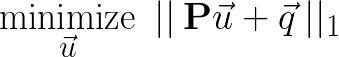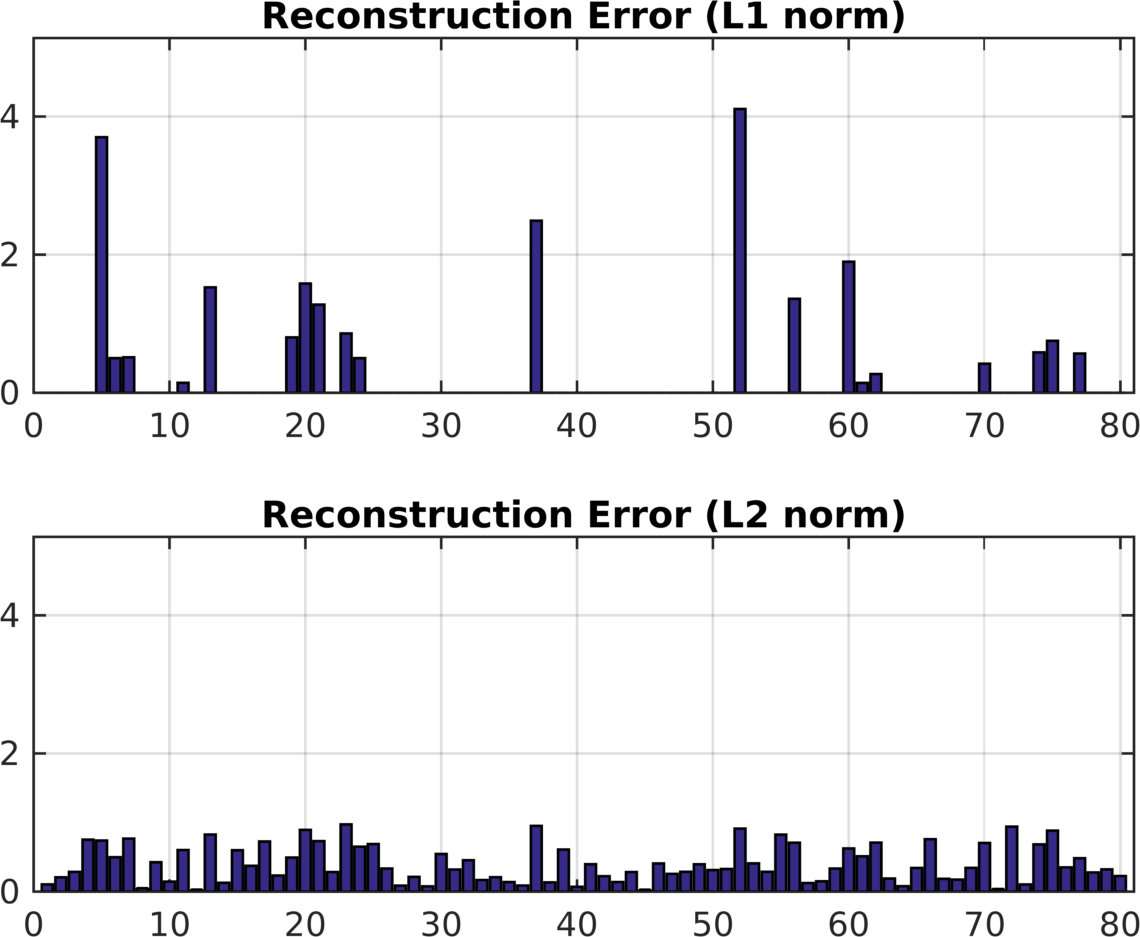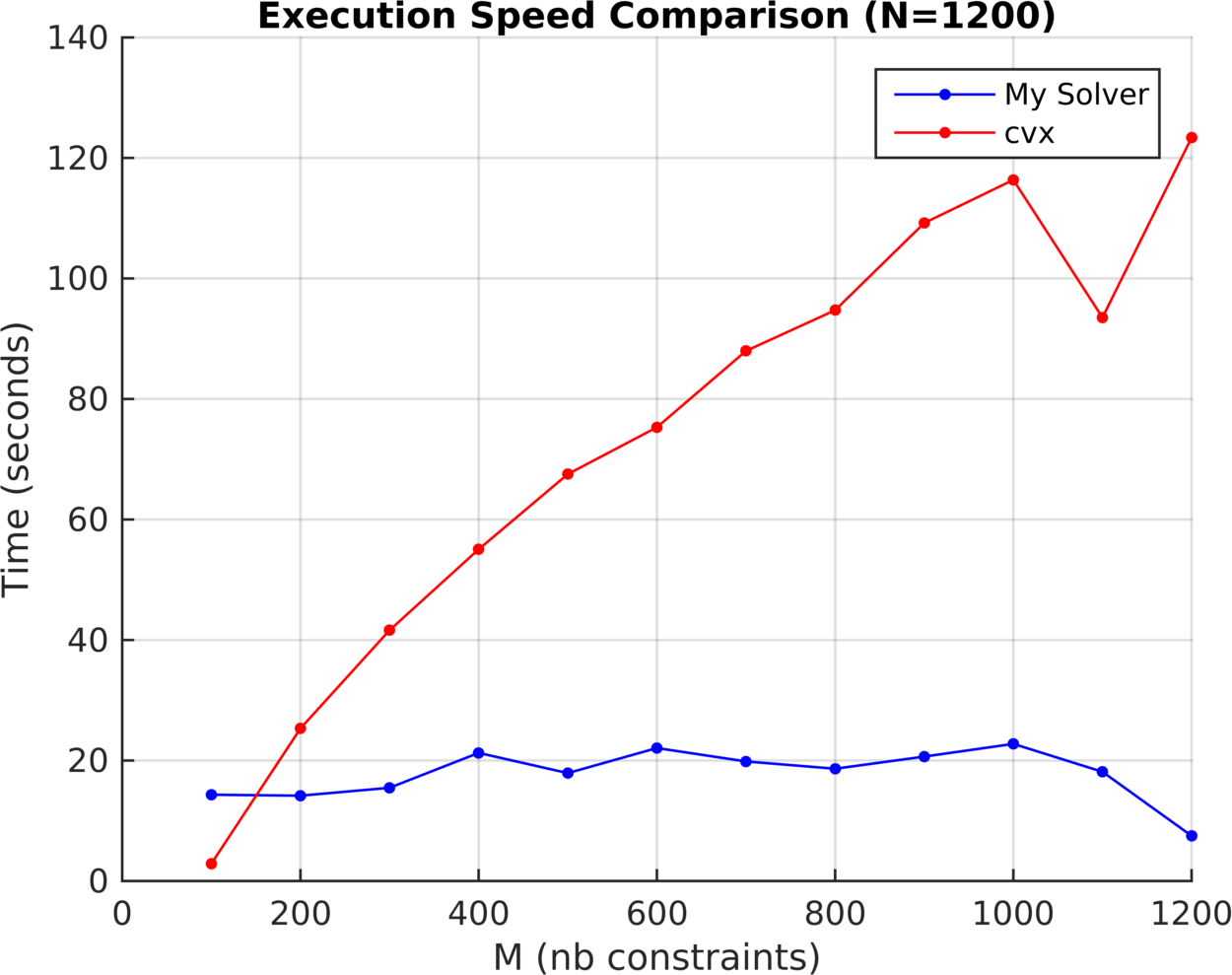# L1-norm Approximation eric-kim.net

This software is a custom solver for the following optimization problem:Where P is a real-valued matrix with m rows and n columns, and both u and q are vectors with n entries.

For medium to large-sized problems (m > 800, n > 200), this software significantly outperforms general-purpose optimization software packages such as cvx in execution speed.

The Matlab source code can be found here: Download.

Note: The software requires cvx, which can be found for free here: Link.

## Motivation

L1-norm approximation is useful compared to L2-norm approximation (ie linear least squares) when you would prefer to perfectly reconstruct as much of the target signal q as possible.

For illustration, consider the following figure that compares L1-norm vs L2-norm approximation for the same problem data (N=60, M=80):Figure 1: Pictured is the reconstruction error abs(P*u + q) for L1-norm and L2-norm approximation respectively.

L1-norm perfectly reconstructs many entries of q, as shown by the many zero-entries in the bar graph. On the other hand, L2-norm spreads out the reconstruction error across all variables such that the overall average error is reduced.

Note that L2-norm achieves a better overall reconstruction. Here, L1-norm gets a 0.6547 mean-square error, whereas L2-norm gets 0.2351. However, depending on your application, you may prefer the L1-norm for its sparse error properties over the L2-norm.

Often times, the L1-norm is associated with sparse coding. In this case, L1-norm approximation encourages sparsity in the reconstruction error. However, this approach does not encourage sparsity in the optimization variable u. See here for a great treatment on L1/L2 regularization that does encourage sparsity on the optimization variables - in particular, ridge regression (L2 regularization) and LASSO (L1 regularization).

## Details

The software uses an iterative primal-dual interior-point method to perform the optimization (Wikipedia, Slides). To improve efficiency for medium to large-sized problems, the software exploits the problem structure to significantly speed up each iteration.

The following figure illustrates that this custom solver scales significantly better than cvx:Figure 2: Solver execution time against problem size. While cvx steadily slows down for large problem sizes, the custom solver maintains good performance.

This was a homework assignment completed for EE 236A: Linear Programming, with Professor Lieven Vandenberghe, Fall 2013. For more information, see: pdf.